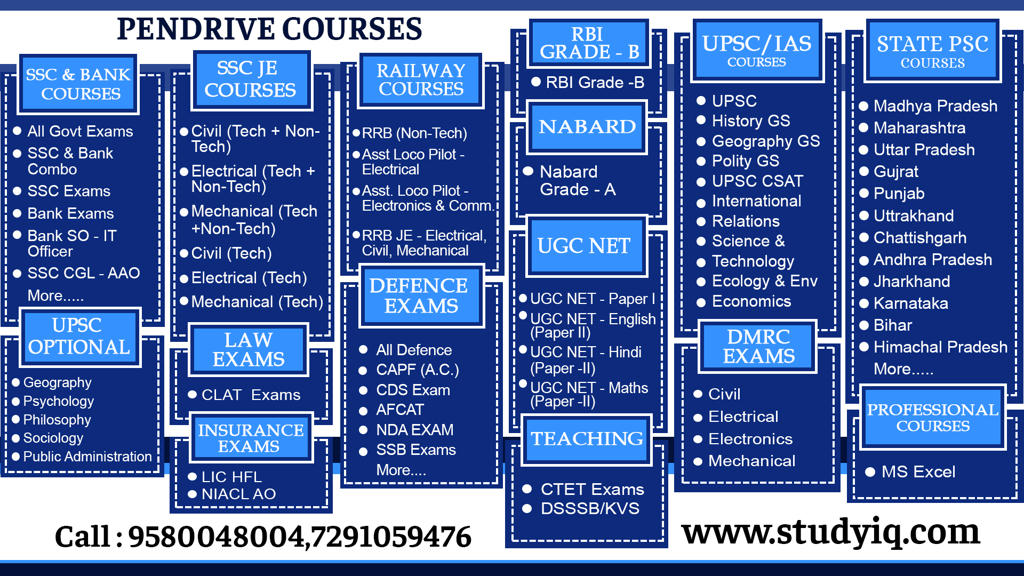# Average Questions – Part 1 – Maths & Reasoning | PDF DownloadQ1. Average of five consecutive number is 27. Find greatest number?

Q2. Average of 7 consecutive number is 20. Find greatest number?

Q3. Average of four consecutive even numbers is 15. Find second highest number in this series

Q4. Average of 7 consecutive numbers is 25. Fin the average of first and last term in this series.

Q5. Find the average of 1 to 50 even numbers .

Q6. Find the average of 1 to 100 odd numbers

Q7. If average of 5 consecutive even numbers is 40 then what is the greatest number.

Q8. Average of 11 consecutive even numbers is 50 then what is the sum of the lowest and highest number.

Q9. Find the average of even numbers between 11 to 63.

Q10. Find the average of even numbers between 51 to 187

Q11. The average of series 40, 43, 46, 49……………….320 is

Q12. Average of 51, 57, 63, 69………………….135 is

Q13. Average of 5 consecutive numbers is n, if two next numbers also taken then what will done in average.

Q14. Average of consecutive natural numbers is m. If next three consecutive numbers also attached then how much more will be the average of all 8 numbers

Q15. Average of 10 number is 12. If 3 is added to each number. Find the new average?

Q16. Average of 10 number is 20. If 5 is subtracted from each number. Find a new average?

Q17. Average of 20 numbers is 25. If each number is multiplied by 2. Find the new average?

Q18. Average of 20 numbers is 50. If each number is divided by 5. Find the new average?

Q19.19 girls and 1 boy go to a restaurant for lunch. If each girl spent Rs. 30 and boy spent Rs. 72000 more than average of expenditure of all. Find the amount spent by the boy?

Q20. 11 girls and 1 boy go to a restaurant for lunch. If each girl spent Rs. 2 and boy spent Rs. 33000 more than average of expenditure of all. Find the amount spent by the boy?